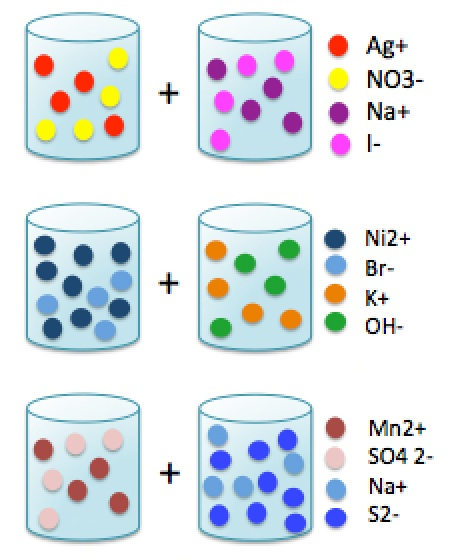# Homework #2

$$\newcommand{\vecs}{\overset { \rightharpoonup} {\mathbf{#1}} }$$ $$\newcommand{\vecd}{\overset{-\!-\!\rightharpoonup}{\vphantom{a}\smash {#1}}}$$$$\newcommand{\id}{\mathrm{id}}$$ $$\newcommand{\Span}{\mathrm{span}}$$ $$\newcommand{\kernel}{\mathrm{null}\,}$$ $$\newcommand{\range}{\mathrm{range}\,}$$ $$\newcommand{\RealPart}{\mathrm{Re}}$$ $$\newcommand{\ImaginaryPart}{\mathrm{Im}}$$ $$\newcommand{\Argument}{\mathrm{Arg}}$$ $$\newcommand{\norm}{\| #1 \|}$$ $$\newcommand{\inner}{\langle #1, #2 \rangle}$$ $$\newcommand{\Span}{\mathrm{span}}$$ $$\newcommand{\id}{\mathrm{id}}$$ $$\newcommand{\Span}{\mathrm{span}}$$ $$\newcommand{\kernel}{\mathrm{null}\,}$$ $$\newcommand{\range}{\mathrm{range}\,}$$ $$\newcommand{\RealPart}{\mathrm{Re}}$$ $$\newcommand{\ImaginaryPart}{\mathrm{Im}}$$ $$\newcommand{\Argument}{\mathrm{Arg}}$$ $$\newcommand{\norm}{\| #1 \|}$$ $$\newcommand{\inner}{\langle #1, #2 \rangle}$$ $$\newcommand{\Span}{\mathrm{span}}$$

Homework is due in class by the date indicated above. Late homework will be docked significantly.

## Q1

Complete the following chemical equations by writing down the correct coefficients.

1. $$Cl_{2\;(g)}+Fe_{(s)} \rightarrow FeCl_{3\;(s)}$$
2. $$Fe_2O_{3\;(s)}+H_{2\;(g)} \rightarrow Fe_{(s)}+H_2O_{(g)}$$
3. $$Al_{(s)}+ O_{2\;(g)} \rightarrow Al_2O_{3\;(s)}$$
4. $$KClO_{3\;(s)} \rightarrow KCl_{(s)}+ O_{2\;(q)}$$
5. $$Al(s) +CuSO_{4\;(aq)} \rightarrow Al_2(SO_4)_{3\;(aq)} +Cu_{(s)}$$

## Q2

Permanganate is the general name for a chemical compound containing the manganate(VII) ion, ($$MnO_4^−$$). Because manganese is in the +7 oxidation state, the permanganate(VII) ion is a strong oxidizing agent. For example, $$KMnO_4$$ will decompose upon heating to produce oxygen (via a disproportionation REOX reaction) via the following unbalanced chemical reaction:

$KMnO_{4\;(s)} \rightarrow K_2MnO_{4\;(s)} + MnO_{2\;(s)} + O_{2\;(g)}$

If 32.5g $$KMnO_4$$ is reacted, what mass of oxygen will be produced?

## Q3

Consider the following reaction.

$Mg(OH)_2 + 2 HCl \rightarrow MgCl_2 + 2 H_2O$

If 25.3 g of $$Mg(OH)_2$$ were reacted with 22.5 g of $$HCl$$. Answer the following questions:

1. What is the limiting reagent?
2. What is the theoretical yield of $$MgCl_2$$?
3. How many grams of excess material are unreacted?
4. If the actual reaction produced 26.6 g of $$MgCl_2$$, calculate the percent yield of generating $$MgCl_2$$.

## Q4

1. Write a balanced molecular equation for the dissociation of $$NaCl$$ in water.
2. Based on the equation in part a, is $$NaCl$$ a strong electrolyte or a weak electrolyte?
3. Hydrofluoric acid is a weak acid, and does not completely dissociate in water. Is it a strong electrolyte or a weak electrolyte?

## Q5

When both of the beakers are added together, chemical reaction takes place. Identify the balanced chemical reaction and the net ionic reaction.## Q6

What are the whole formula equations and the net ionic equations for the following reactions? Write No Reaction if no reactions occur

1. sodium chromate and zinc chloride
2. ammonium nitrate and potassium hydroxide
3. cesium sulfate and mercury(I) nitrate
4. lithium hydroxide and chromium(III) chloride
5. rubidium iodide and sodium bromide

## Q7

If 55.6mL of a 2.0 M solution is diluted to 181.6 mL, what is the final molarity of the solution?

## Q8

Determine concentration of all ions present in the solution when 3.0 g of calcium nitrate is dissolved in water to produce 15.0 mL of solution.

Homework #2 is shared under a not declared license and was authored, remixed, and/or curated by LibreTexts.